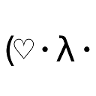# Mort’s small tracesA lifelong project to do my study notes on Math / Computer Science systematically and spirally. It is still woefully incomplete, but it shouldn’t always be. I keep learning things.

“Tiger got to hunt, bird got to fly;
Man got to sit and wonder ‘why, why, why?’
Tiger got to sleep, bird got to land;
Man got to tell himself he understand.”
— Kurt Vonnegut

# 1 Mathematics

• Naive set theory
• Mathematical logic
• Linear algebra
• Matrix theory
• Abstract algebra
• Group theory
• Representation theory
• Category theory and homological algebra
• Topology and geometry
• General topology (point-set topology)
• Geometric topology
• Algebraic topology and geometry
• Differential topology and geometry
• Lie group
• Calculus
• Limit and continuity
• Differentiation
• Integration
• Series
• Special functions
• Vector calculus and tensor calculus
• Calculus of variations
• Analysis
• Measure theory
• Complex analysis
• Real analysis
• Functional analysis
• Harmonic analysis
• Differential equations and dynamical systems theory
• Number theory
• Algebraic number theory
• Analytic number theory
• Combinatorics
• Arithmetic combinatorics
• Graph theory and knot theory
• Algebraic graph theory

# 3 Physics and Life Sciences

• Statistical mechanics
• Classical mechanics, electromagnetism and field theory
• Quantum mechanics and quantum field theory
• Electronics and signal processing
• Formal verification and temporal logic
• Genetics, genomics and bioinformatics
• Structural biology (biochemistry, biophysics and molecular biology)
• Computational systems biology

# 4 Software Technology

• Computer systems and architectures
• Operating systems
• TCP/IP
• Internet protocol suite
• Socket programming
• Web and databases
• Relational database and normalization
• NoSQL
• Knowledge representation and Semantic Web